## Forum Posts

sfolax6776
Sep 29, 2022
In Elementary Math
Find all numbered angles below:0
0
8
sfolax6776
Sep 08, 2022
In Elementary Math
Example 1: #23 of 2007 Practice: #23 of 2008 #19 of 2015
0
0
19
sfolax6776
Jul 18, 2022
In CS Fundamentals for ACSL
1. How many odd vertices are in each of the graphs below? 2. Is the graph below traversable? Can you find an Euler path? 3. Is the graph below traversable? Can you find an Euler path?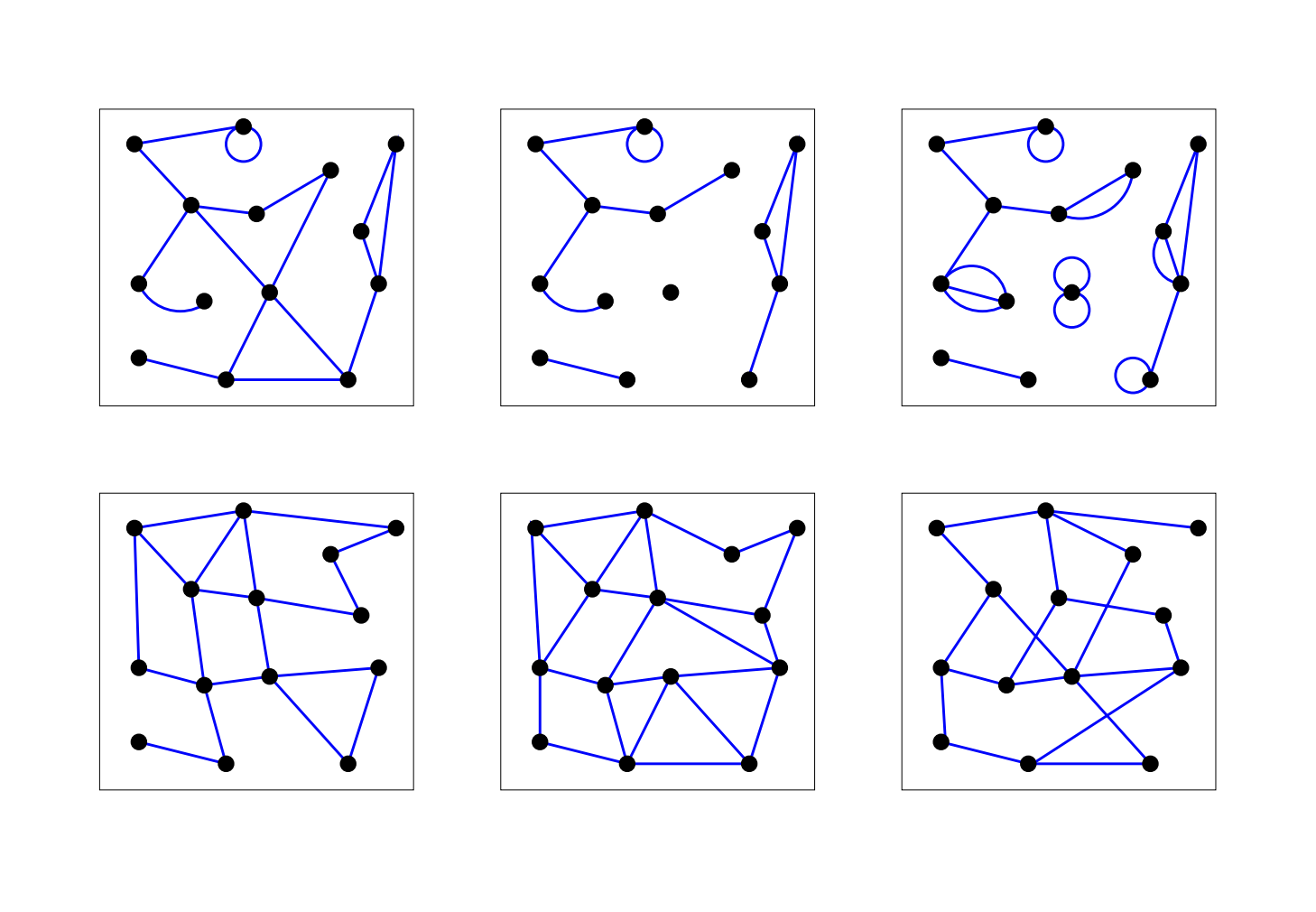0
1
15
sfolax6776
Jun 28, 2022
In Elementary Math
Which turn block(s) will make the pink arrow to point to the strawberry? 2. Which turn block(s) will make the pink arrow to point to the strawberry? 3. What number will you put in the turn block to make the arrow point to the strawberry? the butterfly? the beetle? the apple? Try in Scratch to prove you are correct.0
0
5
sfolax6776
Jun 28, 2022
In CS Fundamentals for ACSL
A. Notes A cycle is a path, which is simple except that the first and last vertex are the same (a path from a point back to itself). ADCBA is a cycle because only the first and last vertex are the same. ABCD is a simple path but not a cycle because the path does not go back to the starting point. CDABEA is a path, but not a simple path because vertex A repeats. It's not a cycle because the starting and ending vertices are not the same. BCDAEB is a cycle because only the starting and ending vertices are the same. Changing only the starting and ending vertex of a cycle will NOT create a new cycle, for example, ABCDA, BCDA, DABC and CDAB are the same cycle, so they count as 1 cycle. The degree of a vertex is the number of edges connected to that vertex. So in the blue graph, vertex A has a degree of 3, vertex B has a degree of 3, vertex C has a degree of 5, and vertex D also has a degree of 3. In 1735 Leonard Euler found that if a graph is traversable, the graph either has no vertex of odd degrees, or has only two vertices of odd degrees. And if a graph has no vertex of odd degrees, you can start at any vertex to traverse the graph; but if the graph has two vertices of odd degrees, you have to start at one odd-degree vertex and end at the other odd-degree vertex. The path to traverse a graph is called a Euler Path. The blue graph has 4 odd-degree vertices, so it is NOT traversable. The black graph above is traversable because it has two odd-degree vertices. B. HW Can you find three different cycles in the blue graph? Can you find more than three? Can you find the Euler Path of the black graph above? Can you find an Euler Path of the graph below?0
1
12
sfolax6776
Jun 21, 2022
In CS Fundamentals for ACSL
A. Notes A path between two vertices in a graph is a list of vertices, in which successive vertices are connected by edges in the graph. The length of a path is the number of edges on the path. A simple path is a path with no vertex repeated. A graph is connected if if there is a path from every vertex to every other vertex in the graph. A graph is traversable if you can draw a path (not simple path) covering all the vertices without retracing any edge. Obviously, a traversable graph is connected. B. HW 1. In the graph below, how many paths can you find from A to D? Is this graph connected? How many simple paths from A to F are there? Is this graph traversable? 2. In the graph below, what is the longest simple path? Is this graph traversable? If you can add an edge, can you make the graph traversable?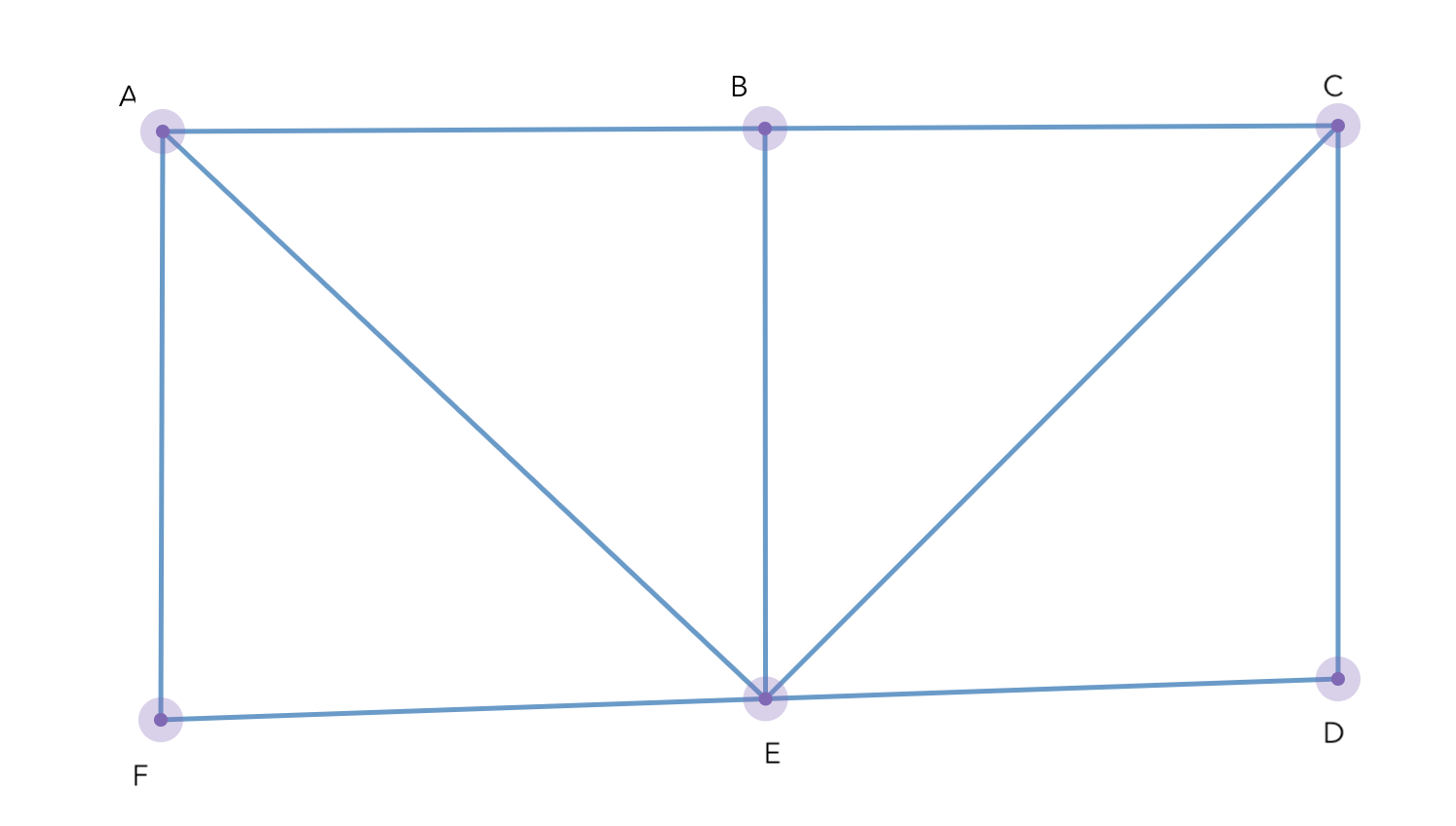0
2
15
sfolax6776
Jun 13, 2022
In CS Fundamentals for ACSL
A. Notes We learned that graphs are made of vertices and edges. We use a set of all vertices and all edges to describe a graph. For the elementary division, we only study undirected graphs. B. HW Draw a graph using the first letters of each person’s name: Alice talks to Bob, Bob talks to Carol, Carol talks to Alice, Alice talks to Doug, and Doug talks to Eve. The graph shows all of the conversations. Draw an undirected graph that has vertices {P, Q, R, S, T} and edges {PQ, RS, ST, PT, QT, RQ, TR}. Can you give a meaning of this graph? Draw a graph to show the cleaning robot's trace according to the description below. Can you think of a different moving strategy to ensure that the robot will pick up all pieces of trash? 4. There are 5 pieces of toys showing below. How many ways are there to rearrange them?0
0
9
sfolax6776
Jun 07, 2022
In CS Fundamentals for ACSL
A. Notes DeMorgan’s Law tells us how to negate a combined statement such as A OR B, and A AND B. NOT (A OR B) = NOT A AND NOT B i.e. ~ ( A + B ) = ~A * ~B NOT (A AND B) = NOT A OR NOT B i.e. ~( A * B) = ~A + ~B Here, A and B can be any statement such as "Noah eats more apples than Justin" or "x < 3" B. HW Practice 1. Let A be " x<3" and B be " x >10" , draw a number line, color all numbers less than 3 red, and all number greater than 10 blue, and the rest yellow. What is the color of a number that is A OR B? What is the color of a number that is NOT(A OR B)? What is the color of a number that is "NOT A AND NOT B"? 2. Let A be " x<3" and B be " x >10" , draw a number line, color all numbers less than 3 red, and all number greater than 10 blue, and the rest yellow. Can you find a number that is A AND B? Can you find a number that is NOT(A AND B)? Can you find a number is "NOT A OR NOT B"? 3. Can you think of another example similar to the above to prove DeMorgan's Law? For instance, you can assume that A is " y > 0" and B is " y < 5". What do ~ ( A + B ) and ~A * ~B mean then? Are they always equivalent? What do ~( A * B) and ~A + ~B mean? Are they always equivalent? 4. Simply the following: ~ (A + B) + ~(A + ~B) ~ (A * B) + ~(A * ~B)
0
2
10
sfolax6776
May 23, 2022
In CS Fundamentals for ACSL
HW: Review all the statements below, are they true or false? X + Y = Y * X X * Y = YX (X + Y) + Z = (Y + Z) + X AC + BC = (A+B)*C A + 0 = 0 A + 1 = A X * 0 = 0 Y * 1 = 1 X + ~Y + Z + 1 = 1 Y ~Y = 0 B + B = B ABC = CAB (D + ~D) ~~X = 1 2. Simplify the following boolean expressions ~A ( B + A~B ) A~B ( A + ~B ) ~A + B~A + ~BA + ~B ~A (B + ~A) + A 3. The question I showed you in the class: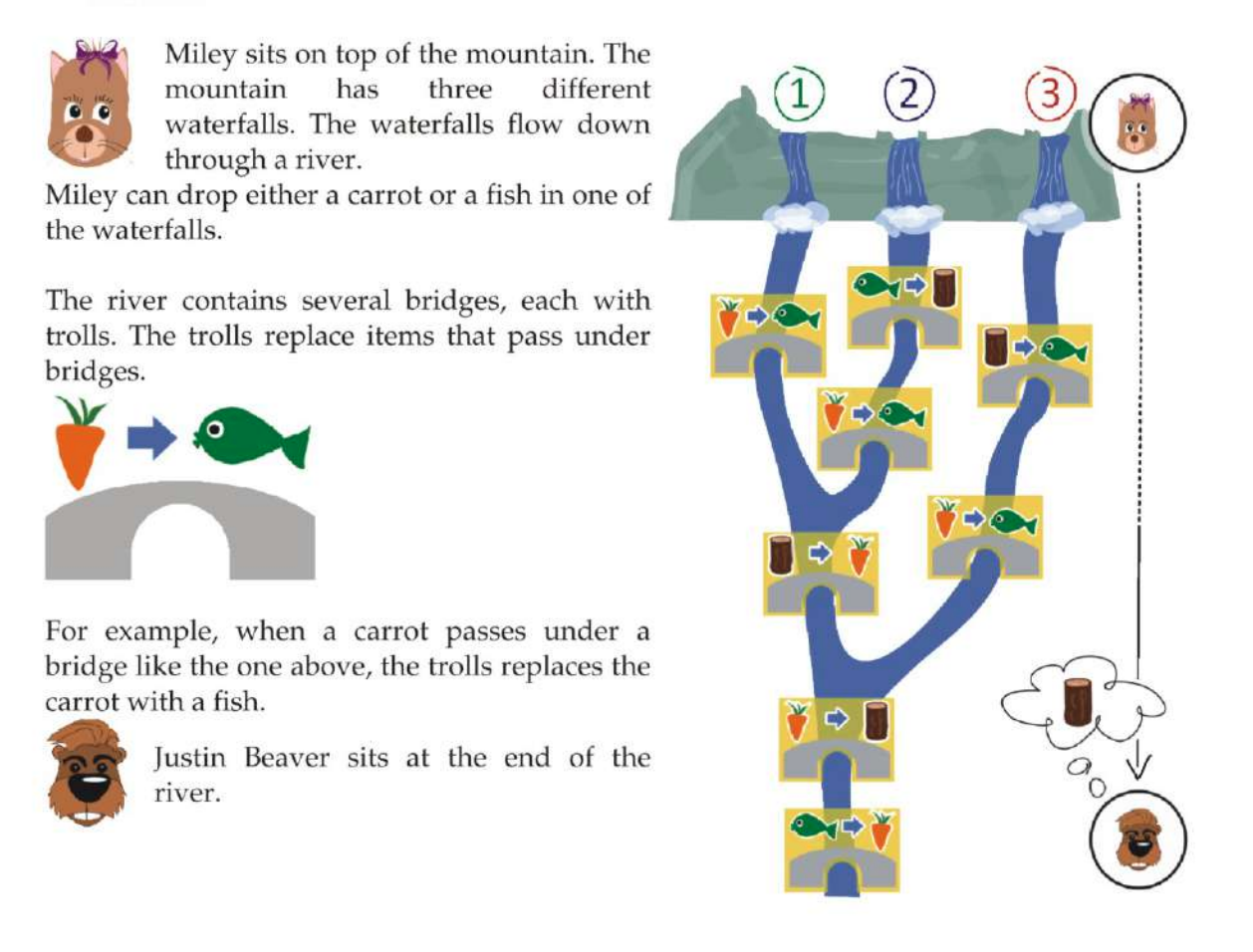0
4
16
sfolax6776
May 09, 2022
In CS Fundamentals for ACSL
A. Notes In this last lesson of boolean algebra, we learned some common identities. Identities refer to the things that are always the same. For instance, A+B and B+A are always the same, so A+B and B+A are identities, we write A+B=B+A. Some commonly used identities in Boolean Algebra are below. #9 above is also called Law of Tautology. We can use these identities to simplify compound boolean expressions. While applying these identities, please keep in mind: A * or a dot both means AND, and sometimes we even omit them. i.e. AB, A*B, A&&B all mean A AND B. A bar on top of a variable means NOT, just as ! and ~. A bar on top of a compound expression means to negate the whole expression. See the example below. Solution I and II both simplify this boolean expression. B. HW Simplify the following boolean expressions: 2. Use DeMorgan's Law to simplify the following:0
2
17
sfolax6776
May 02, 2022
In CS Fundamentals for ACSL
A. Notes Boolean expressions such as !A && !(B && !C) are called compound boolean expressions since !, && or || are used to connect multiple boolean variables. We can build a truth table to find out what ordered pairs or triples will make a compound boolean expression true (or false). When evaluating a compound boolean expression, it's a good practice to evaluate one part at a time. B. HWs 1. What ordered pair(s) will make the following boolean expressions false? A || ! B !(A&&B) || A 2. What ordered triple(s) will make the following boolean expressions true? !A || !B || !C !(A || B || C) !(A && B && C) 3. Assume that both a and b are integers. Will "a > b and b < 0" make the following boolean expression always true? !(a <= b) && (a * b > 0) 4. Given that a, b, and c are integers, consider the boolean expression (a < b) || !((c == a * b) && (c < a)) which of the following will guarantee that the expression is true? (A) c < a is false. (B) c < a is true. (C) a < b is false. (D) c == a * b is true. (E) c == a * b is true, and c < a is true.
0
1
11
sfolax6776
Apr 25, 2022
In CS Fundamentals for ACSL
Truth Tables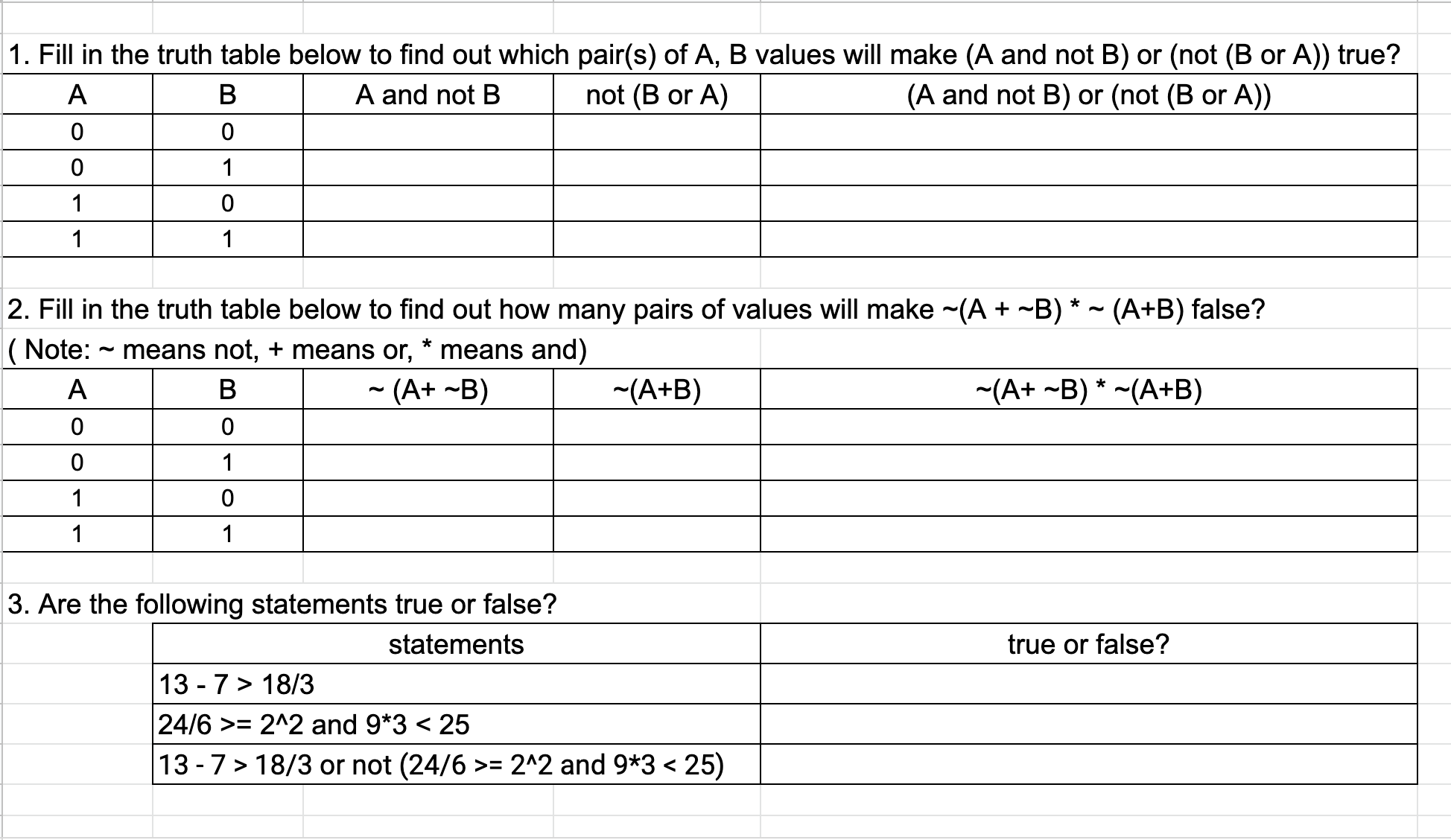0
3
11
sfolax6776
Apr 18, 2022
In CS Fundamentals for ACSL
A. Notes In the computer, we use 1 to represent true and 0 to represent false. We need to use true and false to check facts such as if 100 is greater than 40 We also need and, or, as well as not to connect multiple facts such as "if 100 is greater than 40 or today is the Easter Sunday" We call true and false boolean values. We call and, or, not boolean operators. If we mix boolean values and boolean operators together, we get boolean expressions. B. Exercises0
1
12
sfolax6776
Apr 17, 2022
In AP Computer Science A0
0
4
sfolax6776
Apr 12, 2022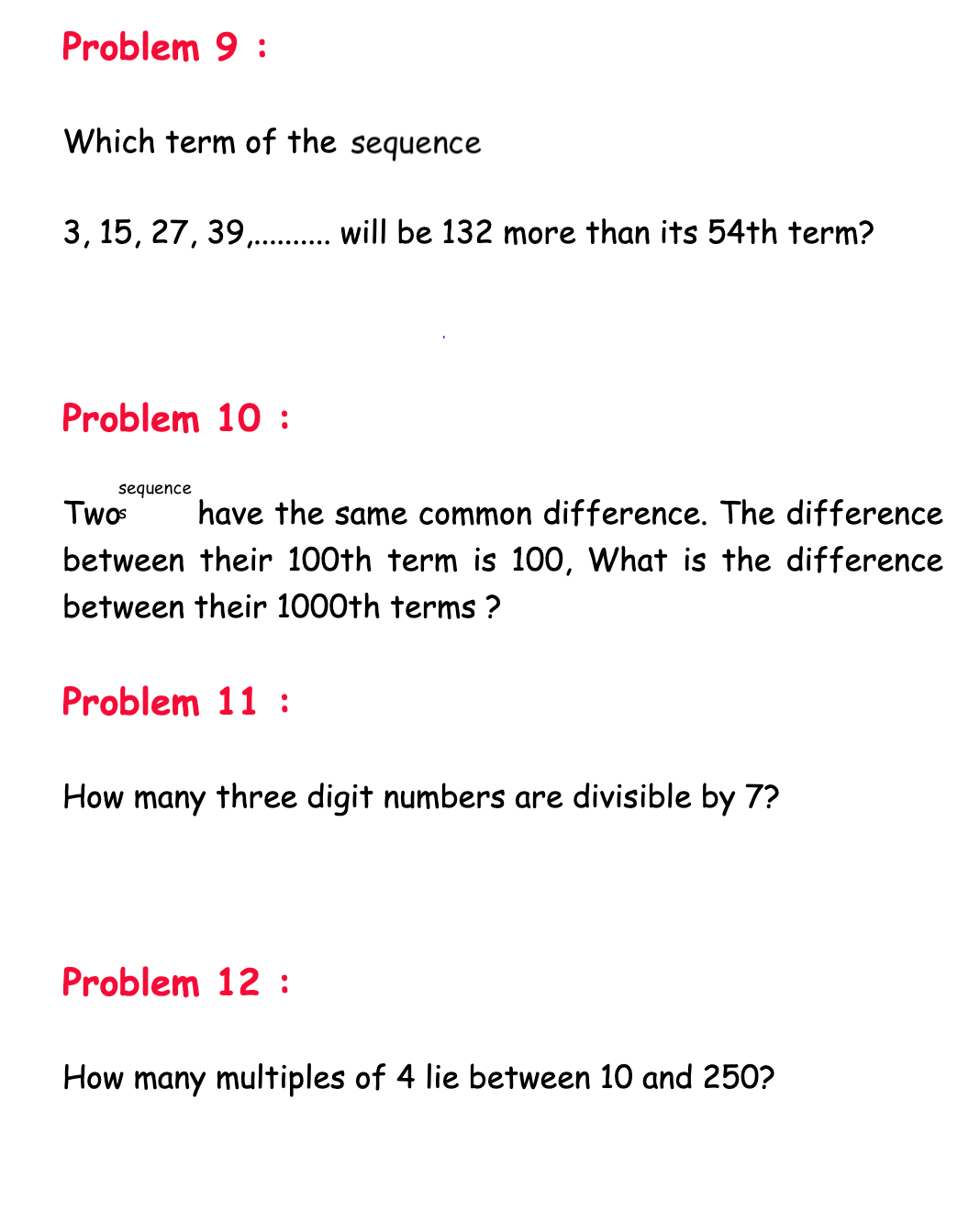0
0
5
sfolax6776
Apr 12, 20220
0
4
sfolax6776
Apr 12, 20220
0
8
sfolax6776
Apr 08, 2022
In Scratch
When the green flag is clicked, a cover screen should show, with background music and a "Next" button When the "Next" button is clicked, an introduction screen should display "how-to play", with the "Next" button, background music continues. When the "Next" button is clicked, all characters show on the stage. With a "little rectangular box with a koala dude (Mr. Buster Moon )in it which looks like a FaceTime icon" inviting the player to click the "Next" button to play a song clip, and guess who the singer is by clicking on the character. When the "Next" button is clicked, the first clip plays. The player can click the character to guess or click on a "Hang up" button to stop the game. If the player guesses correctly, a green check will show and the score increases. Otherwise, a red cross will show and the score decreases. If the player finishes playing all the songs, a "Congrats" screen will show and display the score. If the player clicks "Hang up" before finishing all the songs, a plain black screen will show and display his/her score.
0
1
10
sfolax6776
Apr 04, 2022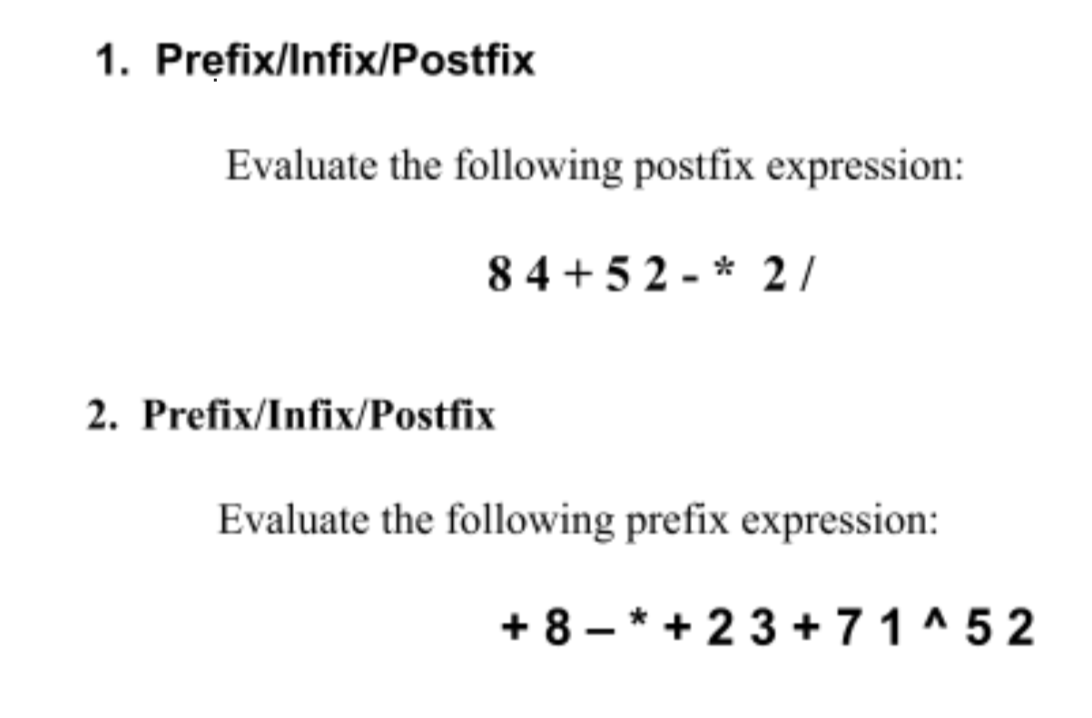0
1
8
sfolax6776
Apr 01, 2022
In AP Computer Science A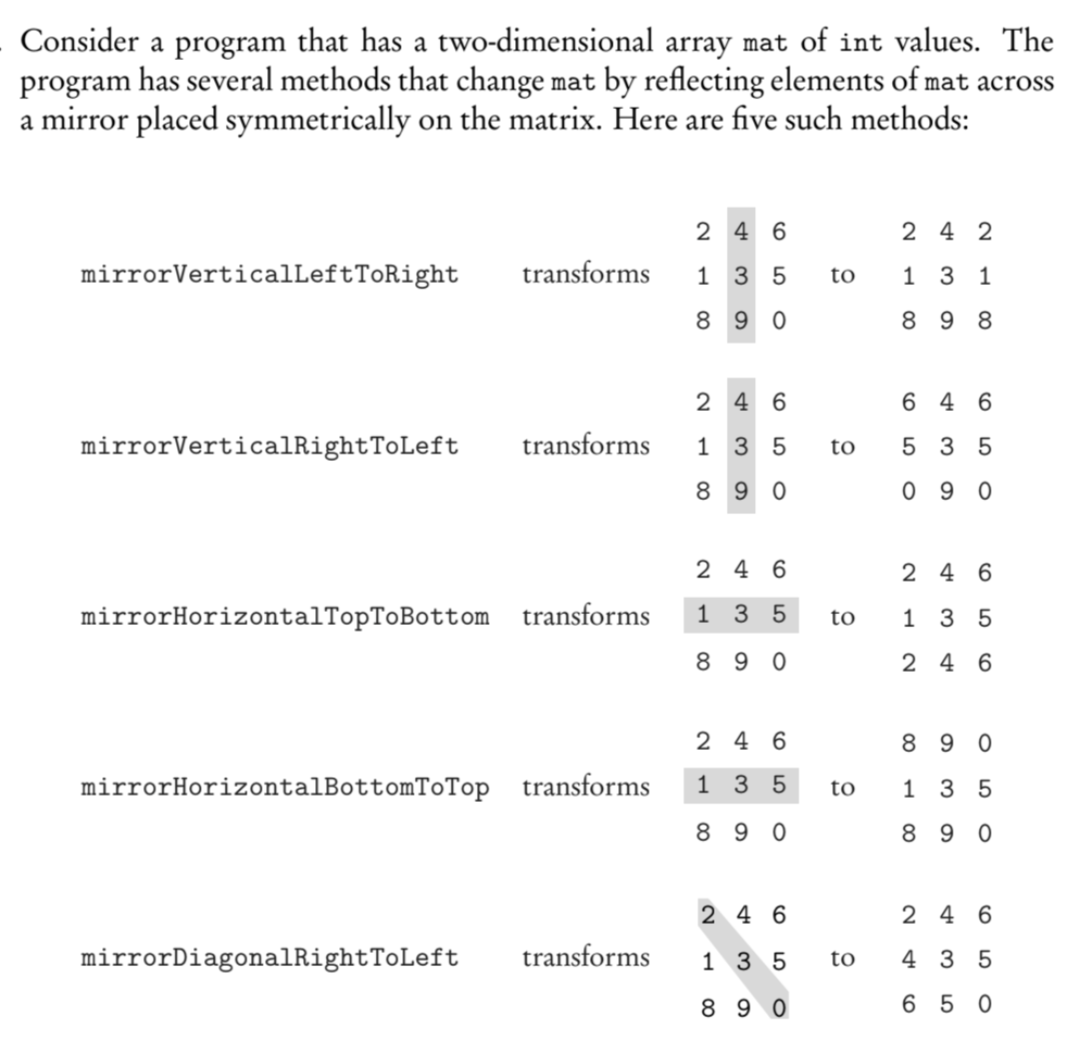0
0
7
S